# Selina Solutions Concise Maths Class 7 Chapter 14: Lines and Angles (Including Construction of Angles) Exercise 14B

Selina Solutions Concise Maths Class 7 Chapter 14 Lines and Angles (Including Construction of Angles) Exercise 14B contains 100% accurate answers, strictly based on the ICSE syllabus. The concept of a transversal, parallel lines and the conditions of parallelism are the topics which are covered under this exercise. The important shortcut tricks and formulas are explained in simple language to impart better understanding among students. Selina Solutions Concise Maths Class 7 Chapter 14 Lines and Angles (Including Construction of Angles) Exercise 14B, PDF can be downloaded from the links available below.

## Selina Solutions Concise Maths Class 7 Chapter 14: Lines and Angles (Including Construction of Angles) Exercise 14B Download PDF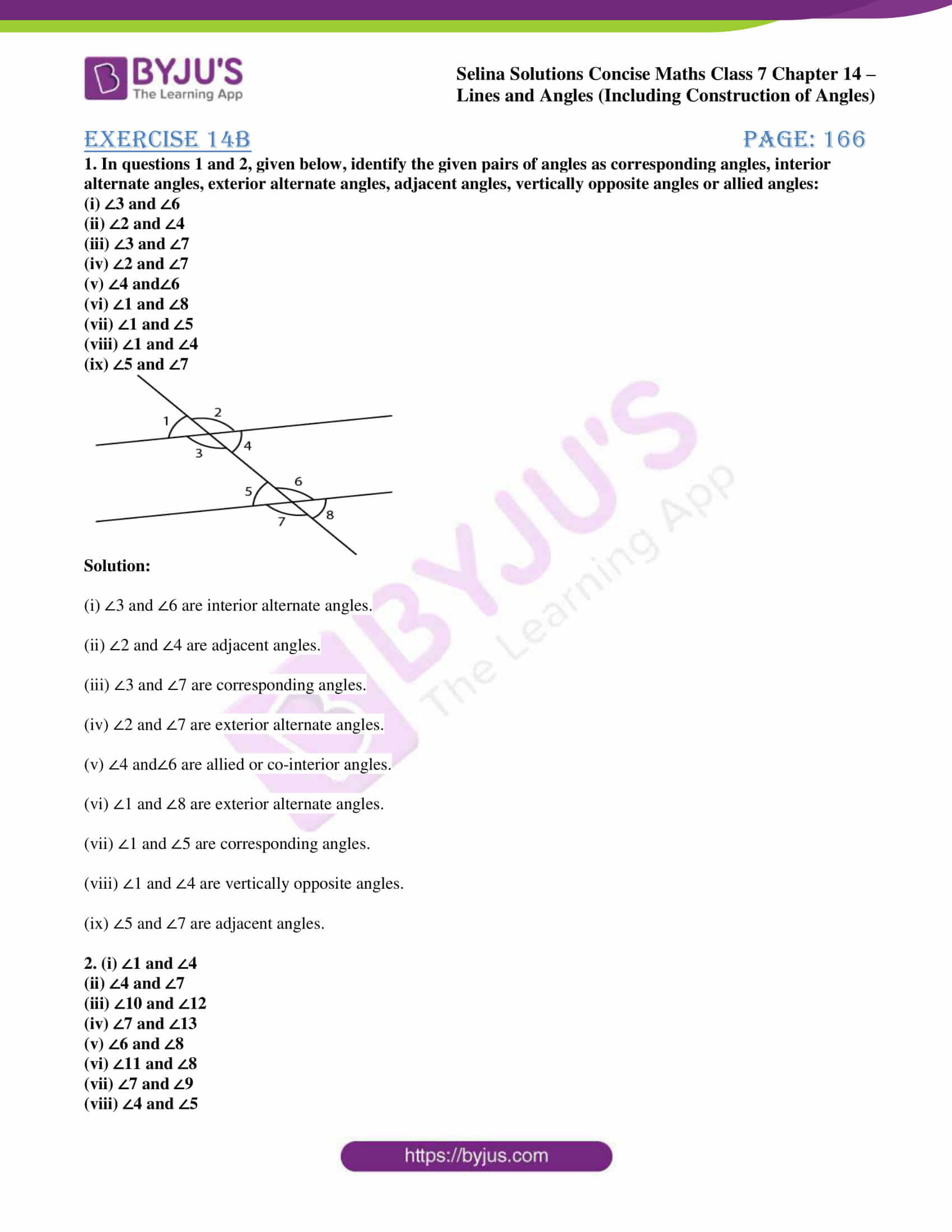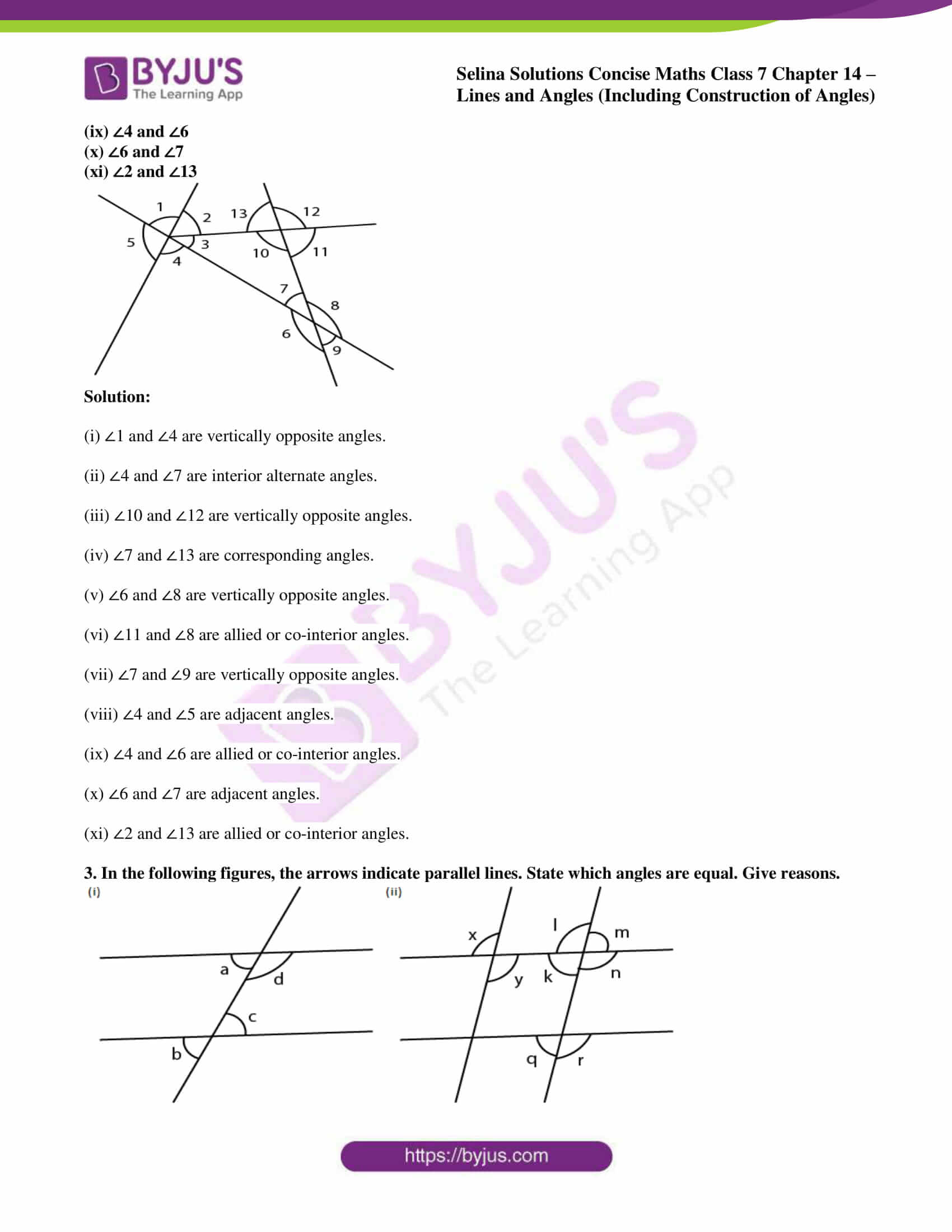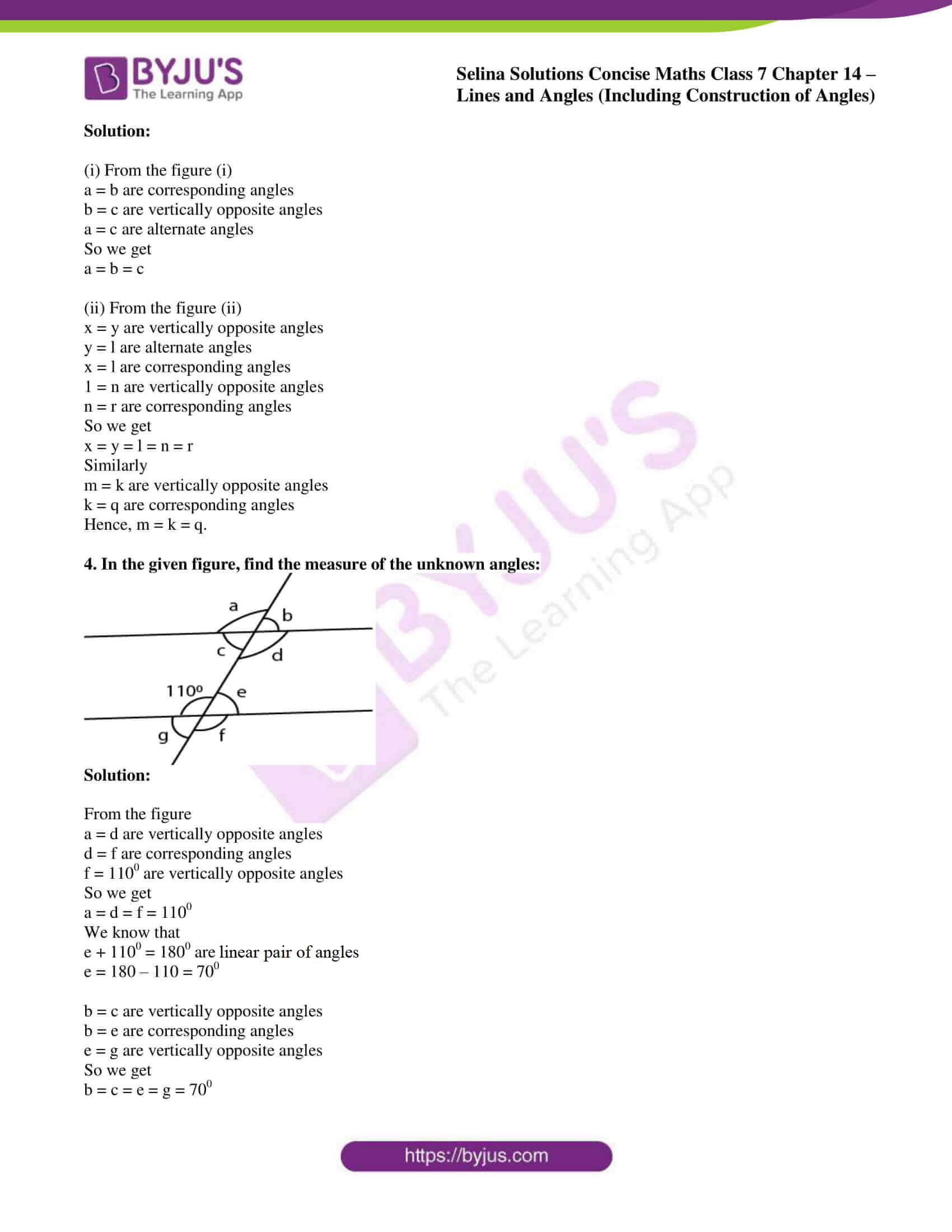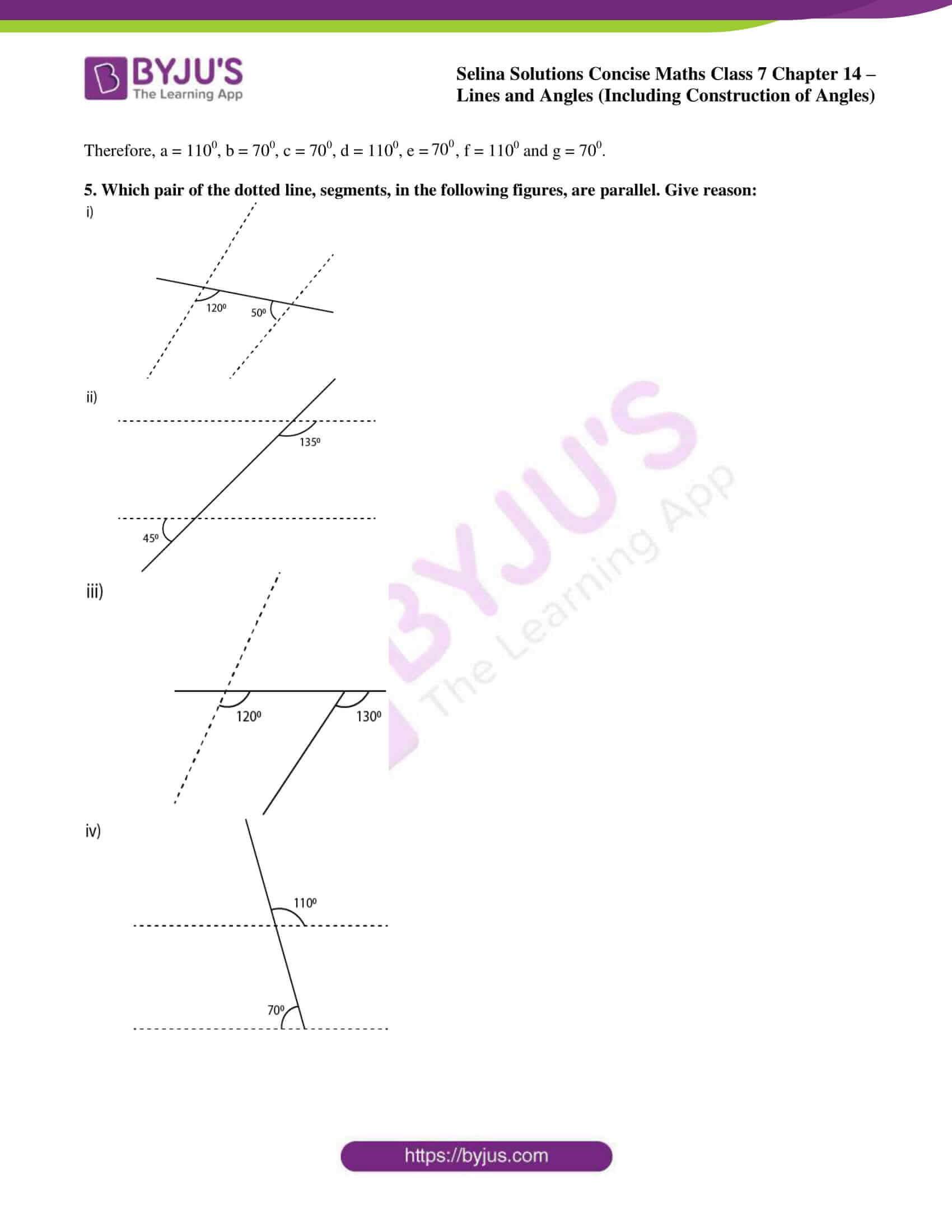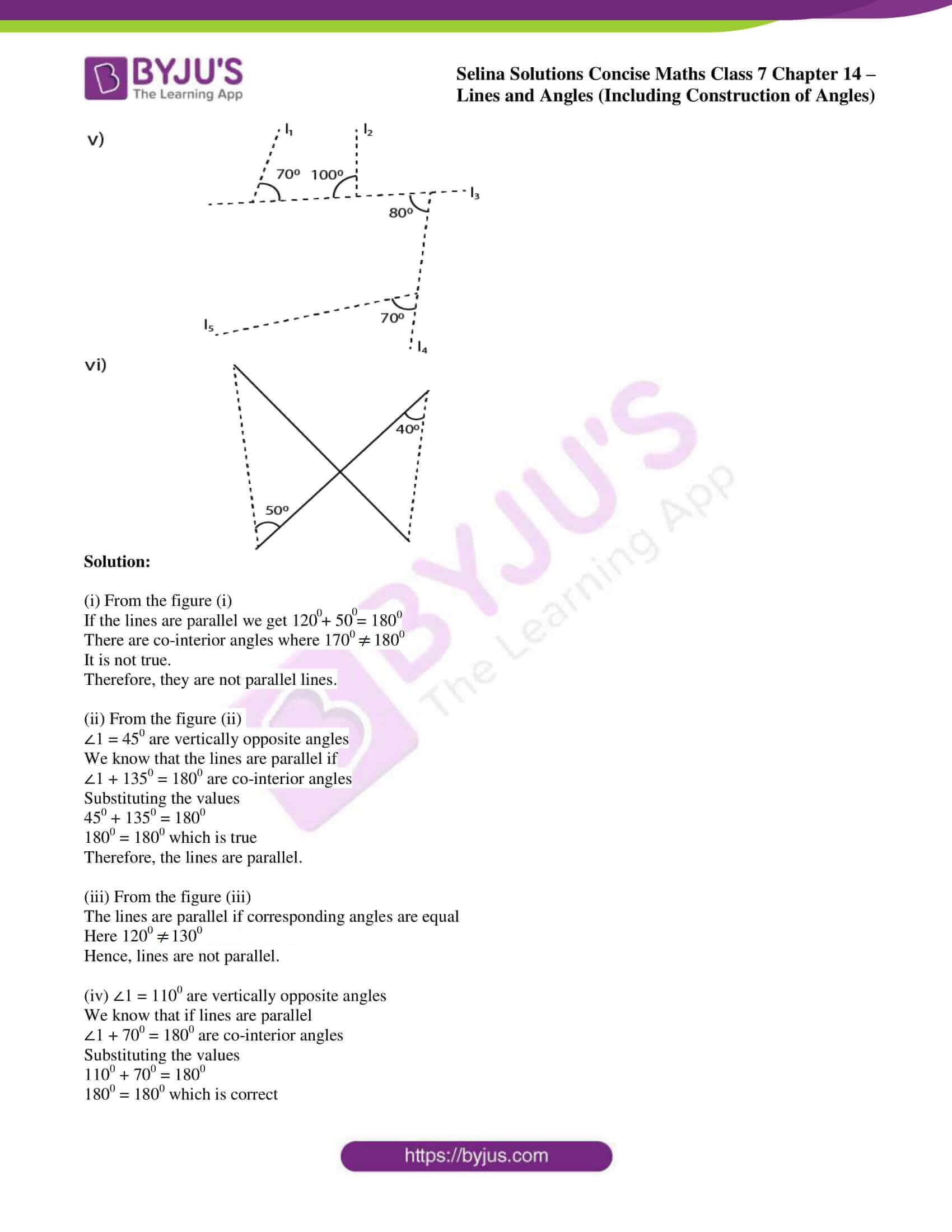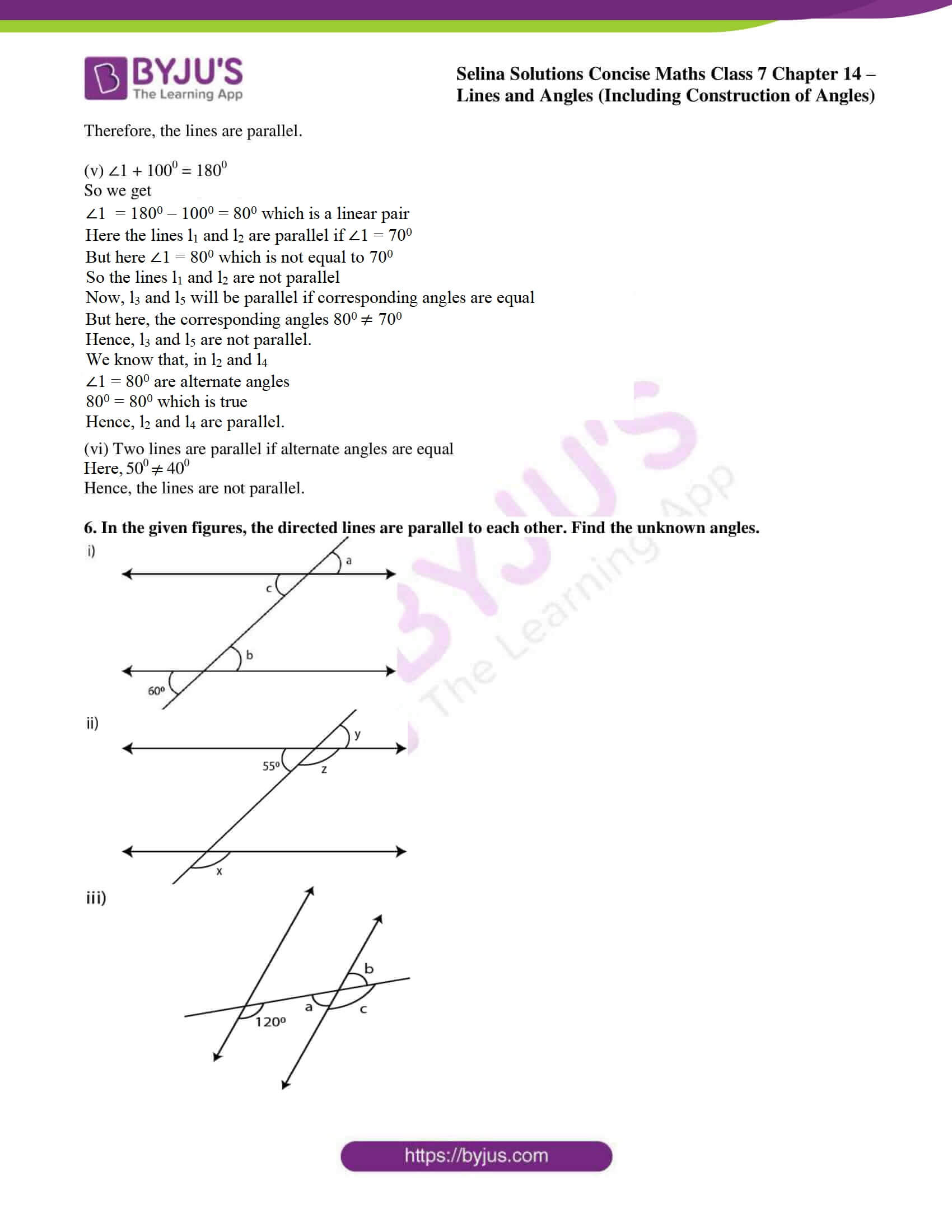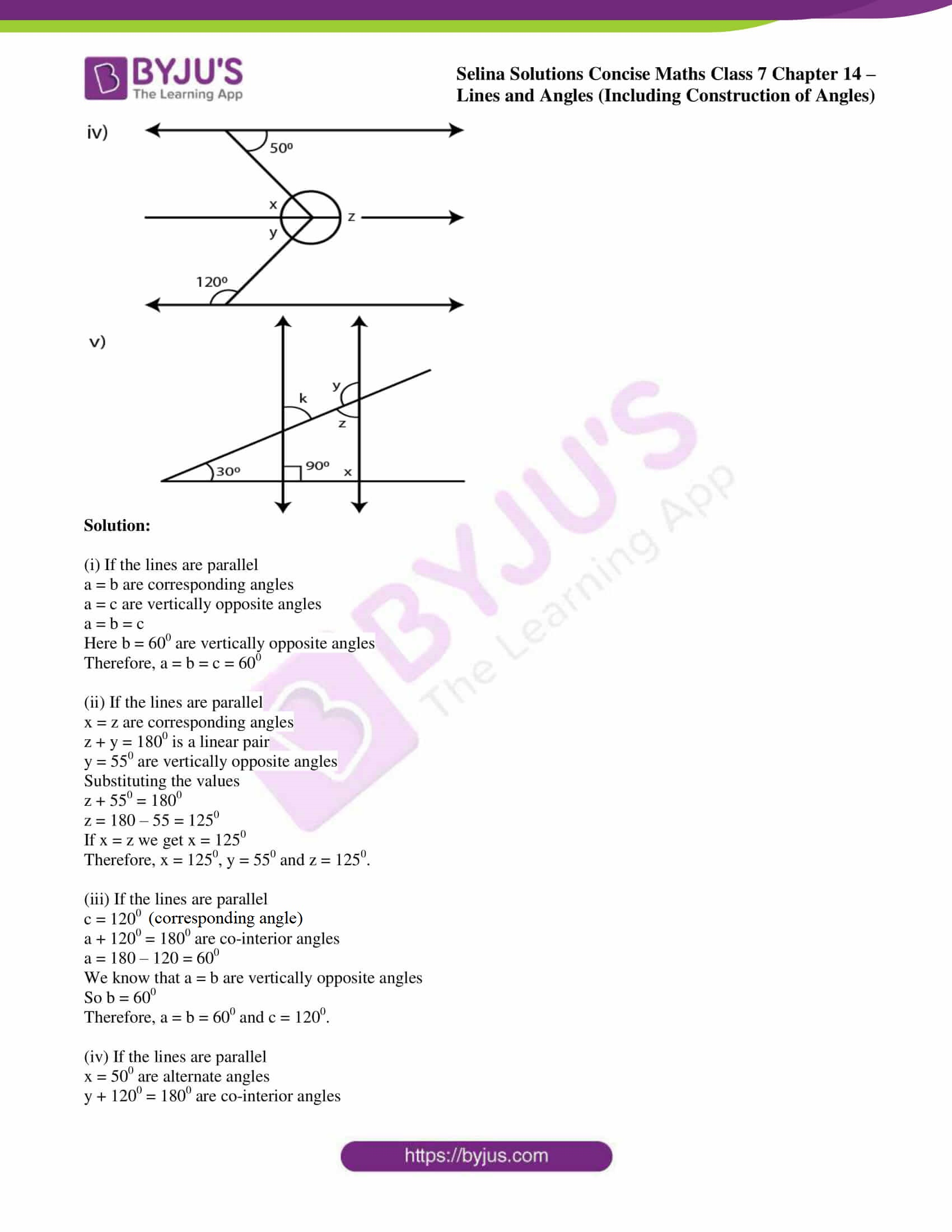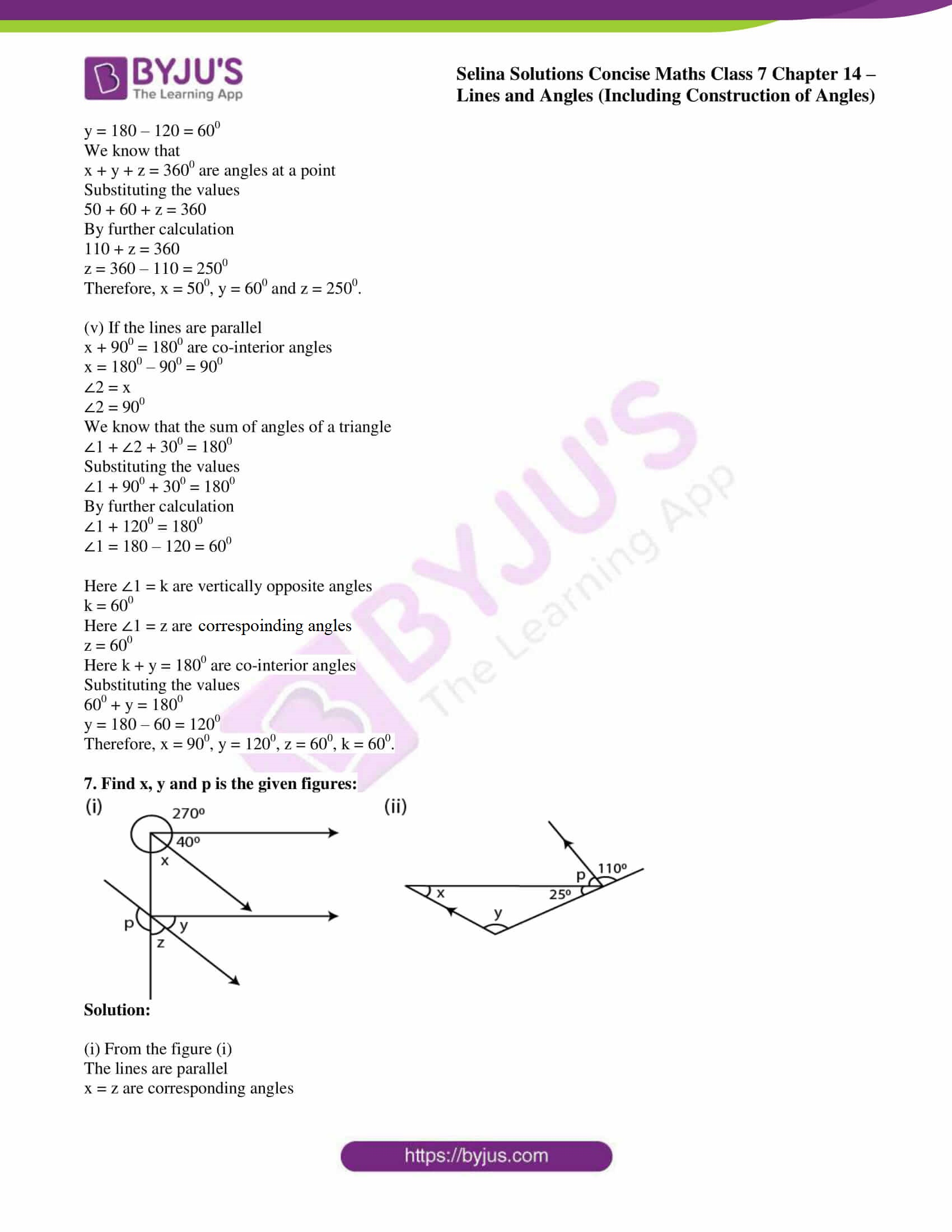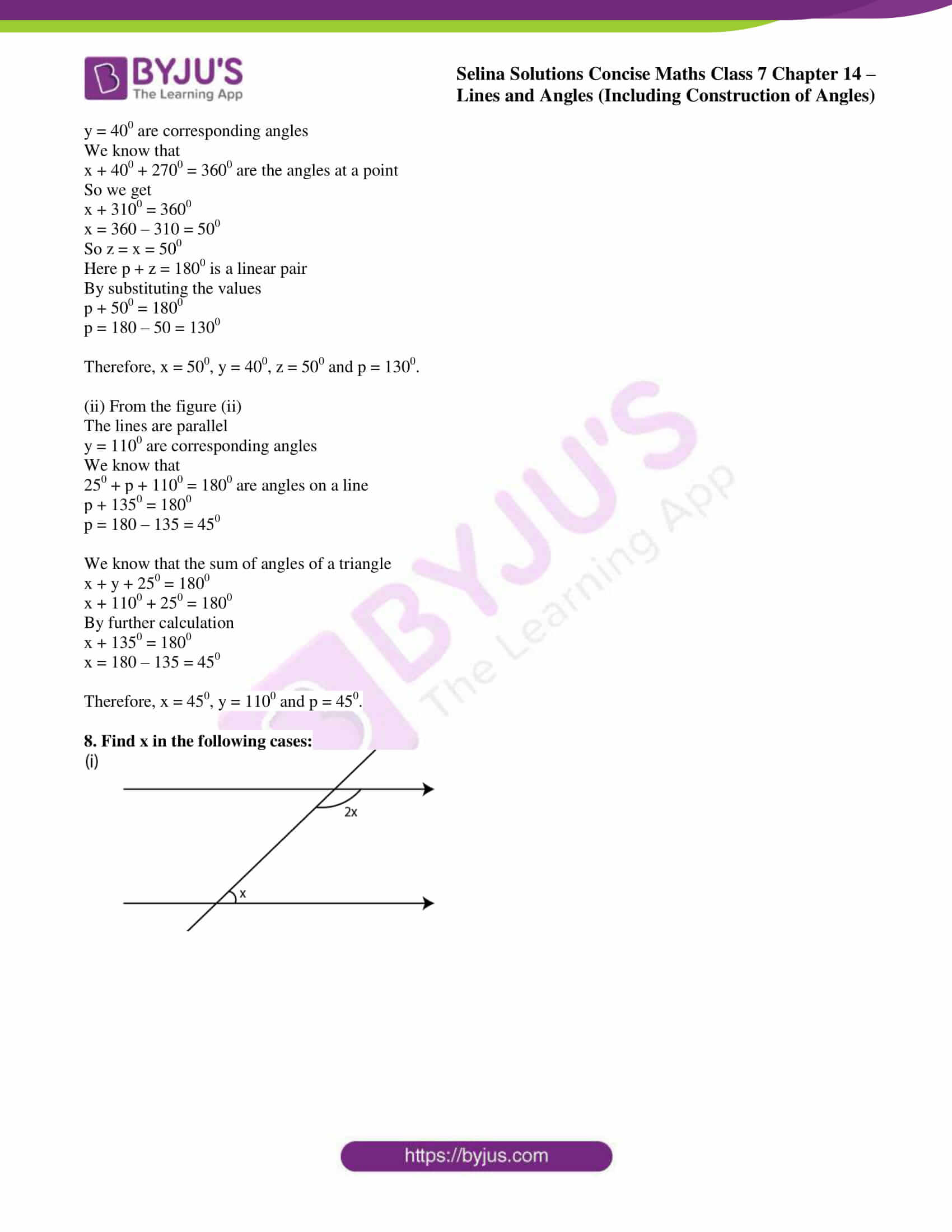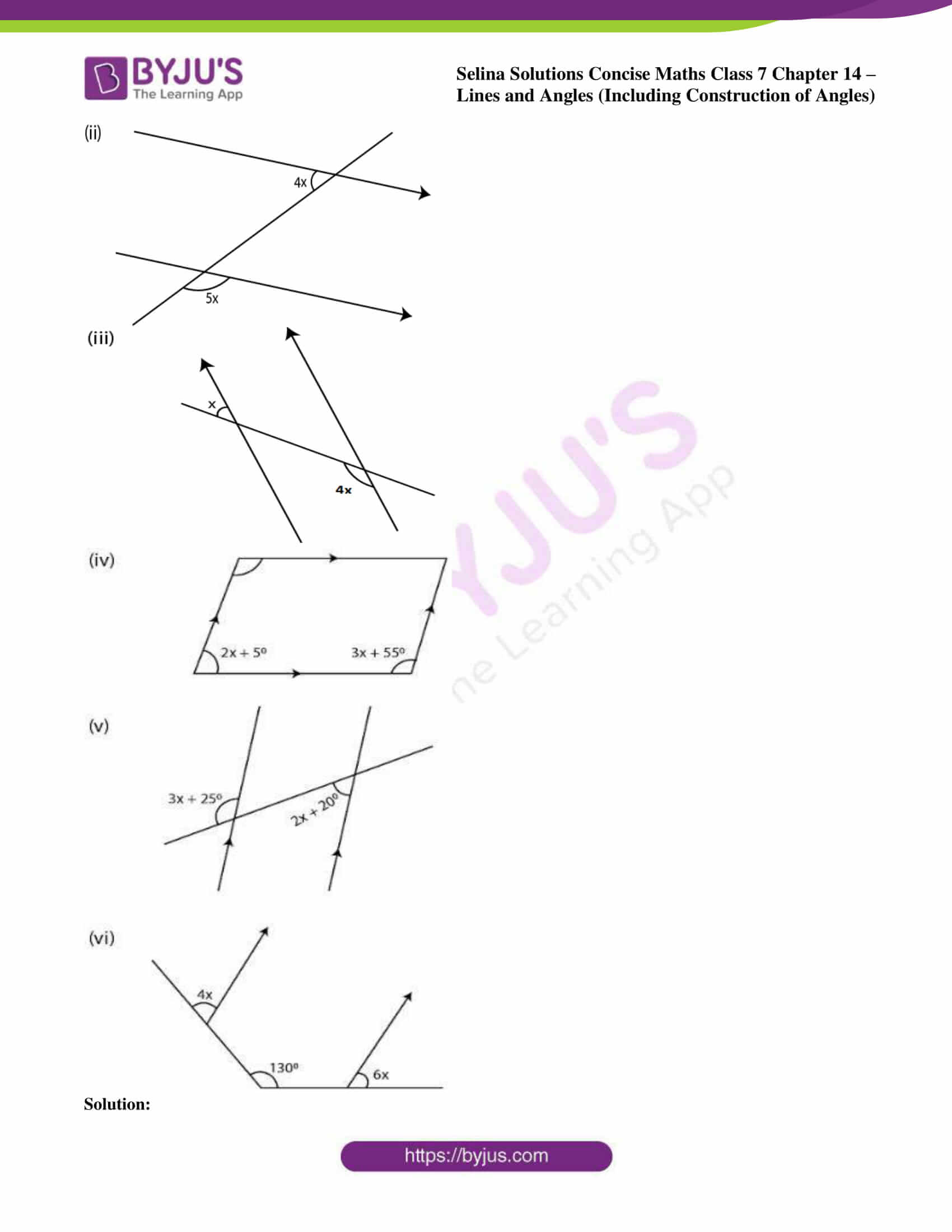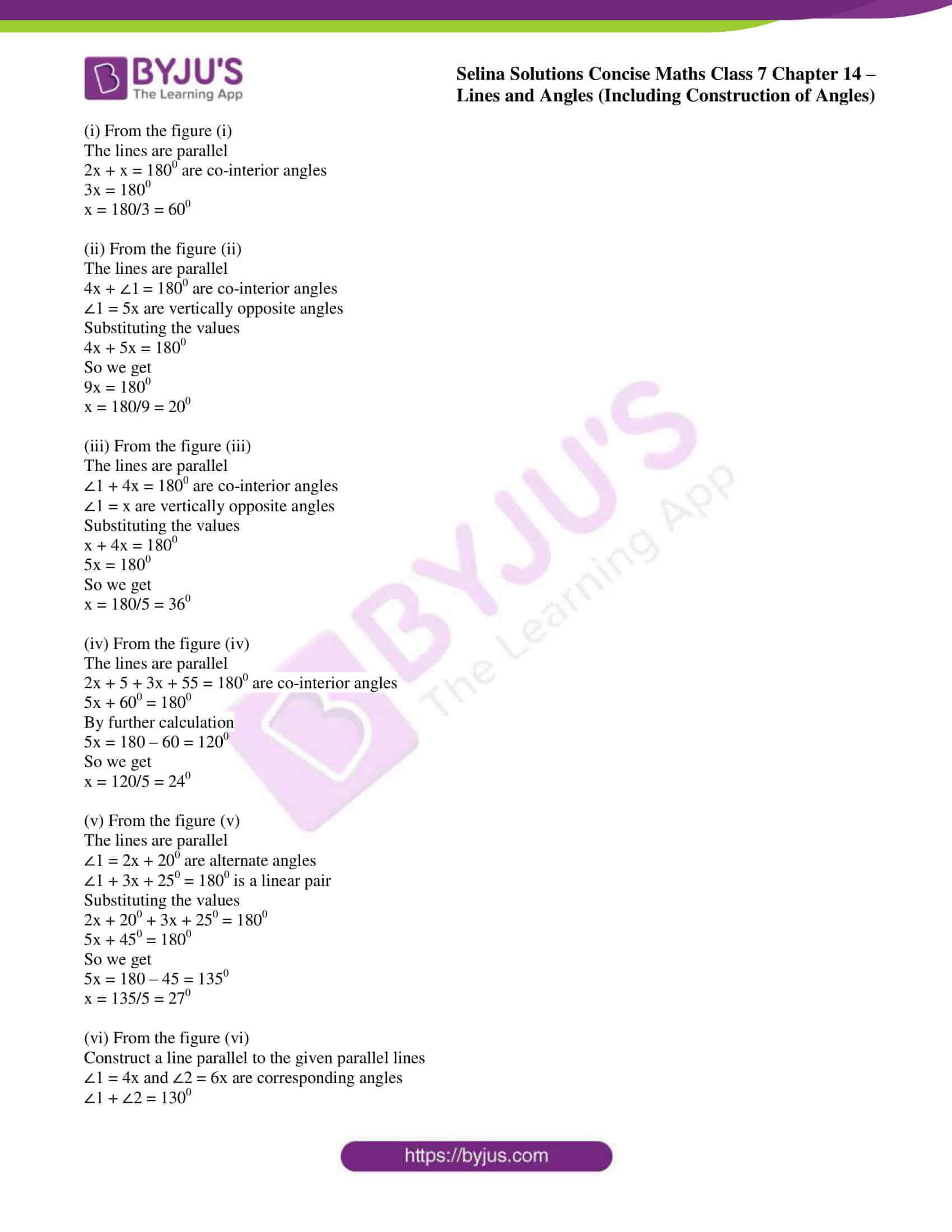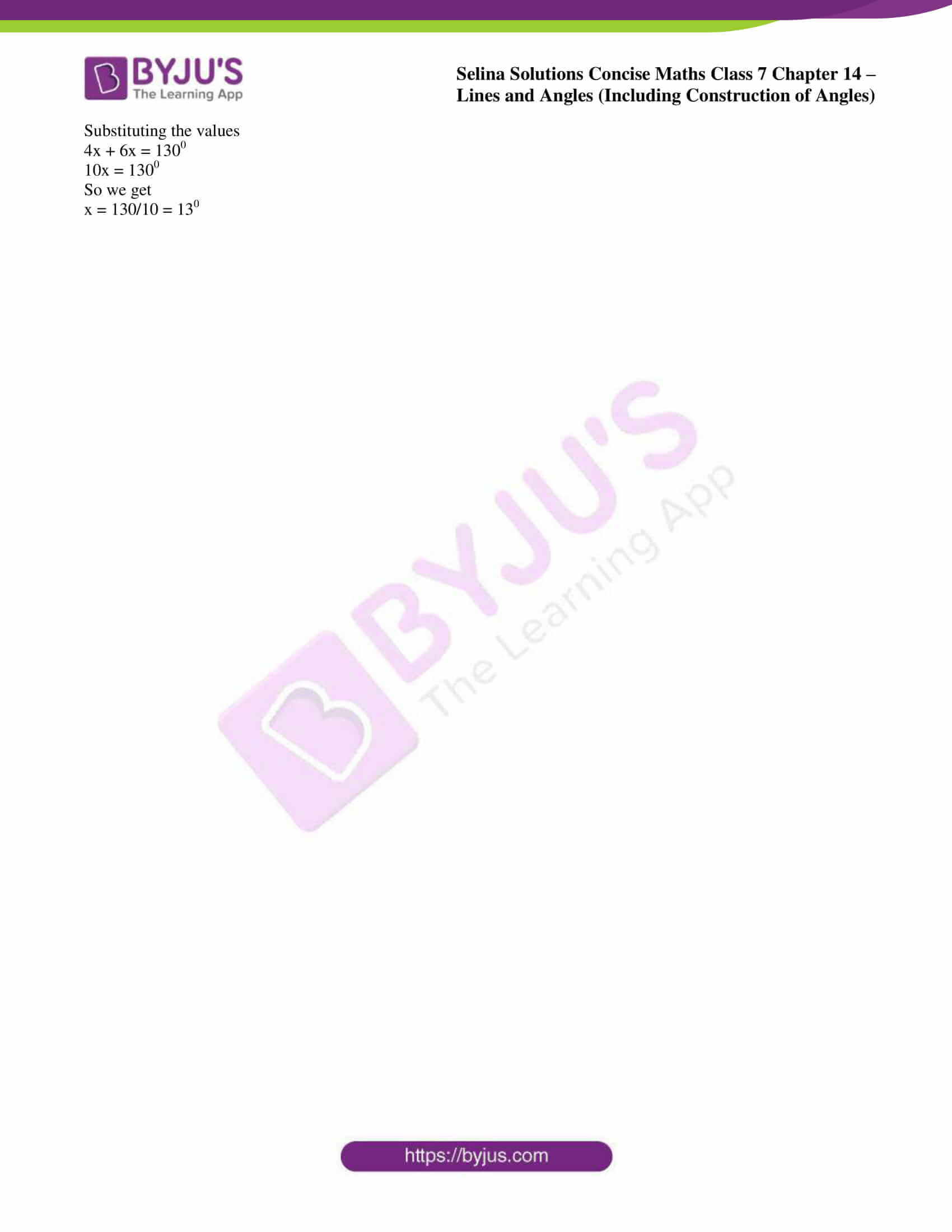### Access other exercises of Selina Solutions Concise Maths Class 7 Chapter 14: Lines and Angles (Including Construction of Angles)

Exercise 14A Solutions

Exercise 14C Solutions

### Access Selina Solutions Concise Maths Class 7 Chapter 14: Lines and Angles (Including Construction of Angles) Exercise 14B

#### Exercise 14B page: 166

1. In questions 1 and 2, given below, identify the given pairs of angles as corresponding angles, interior alternate angles, exterior alternate angles, adjacent angles, vertically opposite angles or allied angles:

(i) ∠3 and ∠6
(ii) ∠2 and ∠4
(iii) ∠3 and ∠7
(iv) ∠2 and ∠7
(v) ∠4 and∠6
(vi) ∠1 and ∠8
(vii) ∠1 and ∠5
(viii) ∠1 and ∠4
(ix) ∠5 and ∠7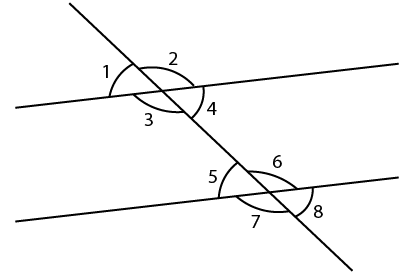Solution:

(i) ∠3 and ∠6 are interior alternate angles.

(ii) ∠2 and ∠4 are adjacent angles.

(iii) ∠3 and ∠7 are corresponding angles.

(iv) ∠2 and ∠7 are exterior alternate angles.

(v) ∠4 and∠6 are allied or co-interior angles.

(vi) ∠1 and ∠8 are exterior alternate angles.

(vii) ∠1 and ∠5 are corresponding angles.

(viii) ∠1 and ∠4 are vertically opposite angles.

(ix) ∠5 and ∠7 are adjacent angles.

2. (i) ∠1 and ∠4
(ii) ∠4 and ∠7
(iii) ∠10 and ∠12
(iv) ∠7 and ∠13
(v) ∠6 and ∠8
(vi) ∠11 and ∠8
(vii) ∠7 and ∠9
(viii) ∠4 and ∠5
(ix) ∠4 and ∠6
(x) ∠6 and ∠7
(xi) ∠2 and ∠13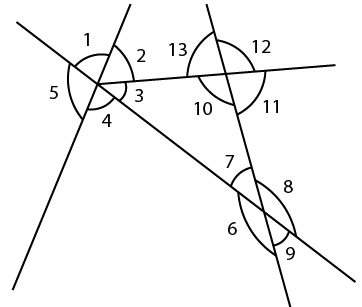Solution:

(i) ∠1 and ∠4 are vertically opposite angles.

(ii) ∠4 and ∠7 are interior alternate angles.

(iii) ∠10 and ∠12 are vertically opposite angles.

(iv) ∠7 and ∠13 are corresponding angles.

(v) ∠6 and ∠8 are vertically opposite angles.

(vi) ∠11 and ∠8 are allied or co-interior angles.

(vii) ∠7 and ∠9 are vertically opposite angles.

(viii) ∠4 and ∠5 are adjacent angles.

(ix) ∠4 and ∠6 are allied or co-interior angles.

(x) ∠6 and ∠7 are adjacent angles.

(xi) ∠2 and ∠13 are allied or co-interior angles.

3. In the following figures, the arrows indicate parallel lines. State which angles are equal. Give reasons.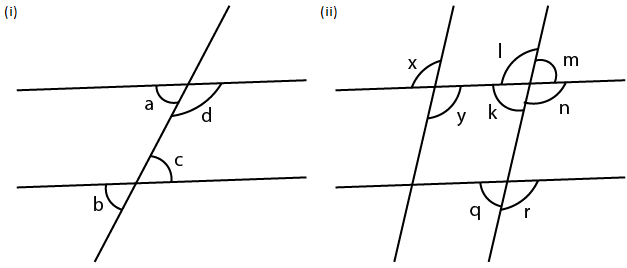Solution:

(i) From the figure (i)

a = b are corresponding angles

b = c are vertically opposite angles

a = c are alternate angles

So we get

a = b = c

(ii) From the figure (ii)

x = y are vertically opposite angles

y = l are alternate angles

x = l are corresponding angles

1 = n are vertically opposite angles

n = r are corresponding angles

So we get

x = y = l = n = r

Similarly

m = k are vertically opposite angles

k = q are corresponding angles

Hence, m = k = q.

4. In the given figure, find the measure of the unknown angles: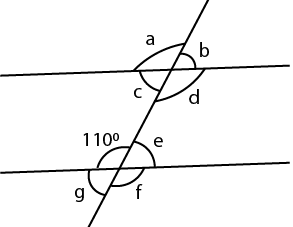Solution:

From the figure

a = d are vertically opposite angles

d = f are corresponding angles

f = 1100 are vertically opposite angles

So we get

a = d = f = 1100

We know that

e + 1100 = 1800 are linear pair of angles

e = 180 – 110 = 700

b = c are vertically opposite angles

b = e are corresponding angles

e = g are vertically opposite angles

So we get

b = c = e = g = 700

Therefore, a = 1100, b = 700, c = 700, d = 1100, e = 700, f = 1100 and g = 700.

5. Which pair of the dotted line, segments, in the following figures, are parallel. Give reason: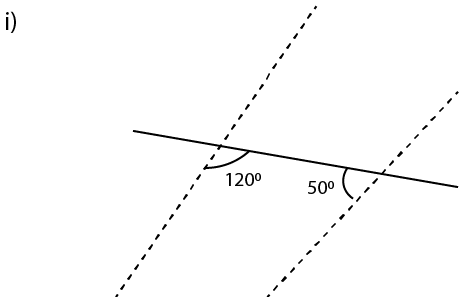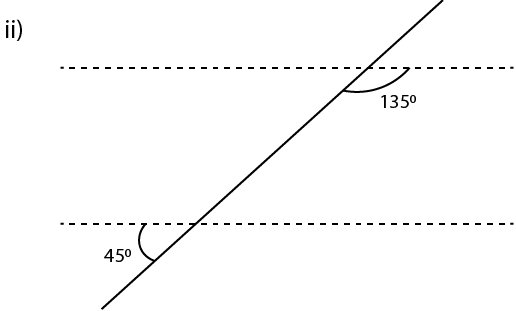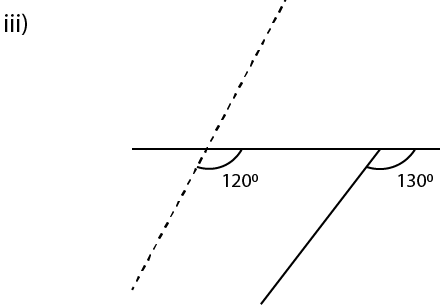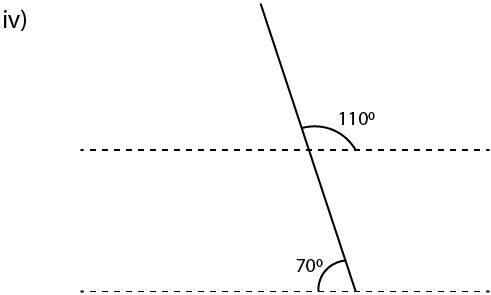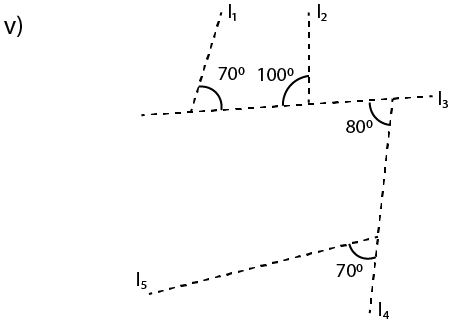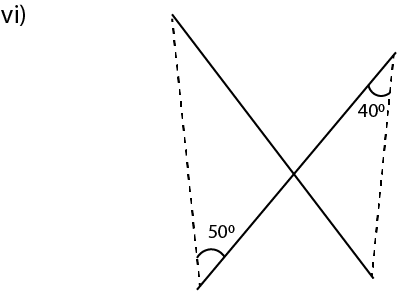Solution:

(i) From the figure (i)

If the lines are parallel we get 1200 + 500 = 1800

There are co-interior angles where 1700 ≠ 1800

Therefore, they are not parallel lines.

(ii) From the figure (ii)

∠1 = 450 are vertically opposite angles

We know that the lines are parallel if

∠1 + 1350 = 1800 are co-interior angles

Substituting the values

450 + 1350 = 1800

1800 = 1800 which is true

Therefore, the lines are parallel.

(iii) From the figure (iii)

The lines are parallel if corresponding angles are equal

Here 1200 ≠ 1300

Hence, lines are not parallel.

(iv) ∠1 = 1100 are vertically opposite angles

We know that if lines are parallel

∠1 + 700 = 1800 are co-interior angles

Substituting the values

1100 + 700 = 1800

1800 = 1800 which is correct

Therefore, the lines are parallel.

(v) ∠1 + 1000 = 1800

So we get

∠1 = 1800 – 1000 = 800 which is a linear pair
Here the lines l1 and l2 are parallel if ∠1 = 700
But here ∠1 = 800 which is not equal to 700
So the lines l1 and l2 are not parallel
Now, l3 and l5 will be parallel if corresponding angles are equal
But here, the corresponding angles 800 ≠ 700
Hence, l3 and l5 are not parallel.
We know that, in l2 and l4
∠1 = 800 are alternate angles
800 = 800 which is true
Hence, l2 and l4 are parallel.

(vi) Two lines are parallel if alternate angles are equal

Here, 500 ≠ 400 which is not true

Hence, the lines are not parallel.

6. In the given figures, the directed lines are parallel to each other. Find the unknown angles.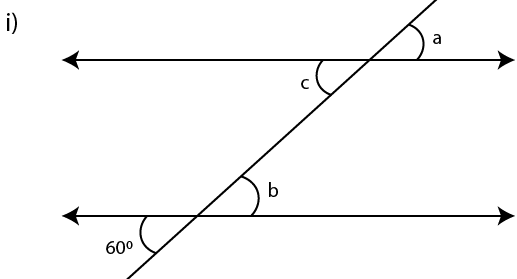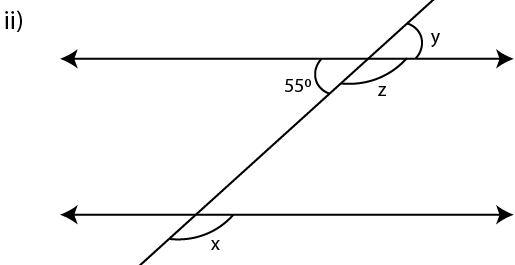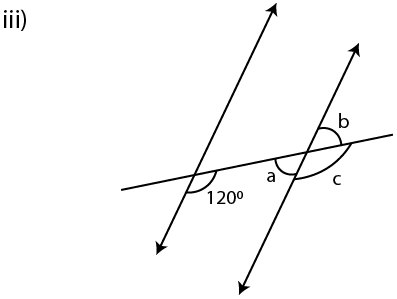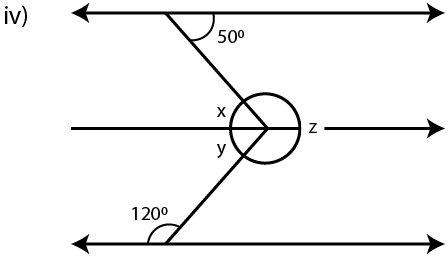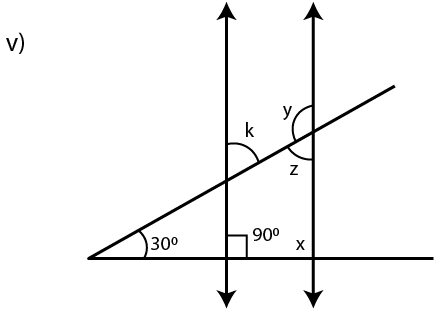Solution:

(i) If the lines are parallel

a = b are corresponding angles

a = c are vertically opposite angles

a = b = c

Here b = 600 are vertically opposite angles

Therefore, a = b = c = 600

(ii) If the lines are parallel

x = z are corresponding angles

z + y = 1800 is a linear pair

y = 550 are vertically opposite angles

Substituting the values

z + 550 = 1800

z = 180 – 55 = 1250

If x = z we get x = 1250

Therefore, x = 1250, y = 550 and z = 1250.

(iii) If the lines are parallel

c = 1200 (corresponding angles)

a + 1200 = 1800 are co-interior angles

a = 180 – 120 = 600

We know that a = b are vertically opposite angles

So b = 600

Therefore, a = b = 600 and c = 1200.

(iv) If the lines are parallel

x = 500 are alternate angles

y + 1200 = 1800 are co-interior angles

y = 180 – 120 = 600

We know that

x + y + z = 3600 are angles at a point

Substituting the values

50 + 60 + z = 360

By further calculation

110 + z = 360

z = 360 – 110 = 2500

Therefore, x = 500, y = 600 and z = 2500.

(v) If the lines are parallel

x + 900 = 1800 are co-interior angles

x = 1800 – 900 = 900

∠2 = x

∠2 = 900

We know that the sum of angles of a triangle

∠1 + ∠2 + 300 = 1800

Substituting the values

∠1 + 900 + 300 = 1800

By further calculation

∠1 + 1200 = 1800

∠1 = 180 – 120 = 600

Here ∠1 = k are vertically opposite angles

k = 600

Here ∠1 = z are corresponding angles

z = 600

Here k + y = 1800 are co-interior angles

Substituting the values

600 + y = 1800

y = 180 – 60 = 1200

Therefore, x = 900, y = 1200, z = 600, k = 600.

7. Find x, y and p is the given figures: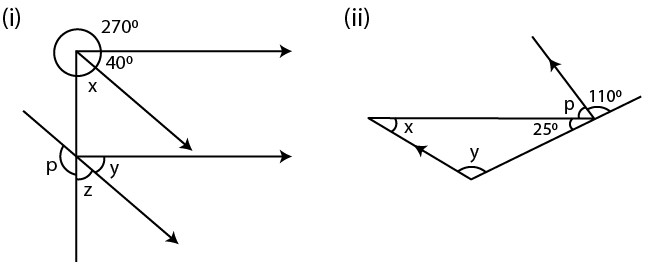Solution:

(i) From the figure (i)

The lines are parallel

x = z are corresponding angles

y = 400 are corresponding angles

We know that

x + 400 + 2700 = 3600 are the angles at a point

So we get

x + 3100 = 3600

x = 360 – 310 = 500

So z = x = 500

Here p + z = 1800 is a linear pair

By substituting the values

p + 500 = 1800

p = 180 – 50 = 1300

Therefore, x = 500, y = 400, z = 500 and p = 1300.

(ii) From the figure (ii)

The lines are parallel

y = 1100 are corresponding angles

We know that

250 + p + 1100 = 1800 are angles on a line

p + 1350 = 1800

p = 180 – 135 = 450

We know that the sum of angles of a triangle

x + y + 250 = 1800

x + 1100 + 250 = 1800

By further calculation

x + 1350 = 1800

x = 180 – 135 = 450

Therefore, x = 450, y = 1100 and p = 450.

8. Find x in the following cases: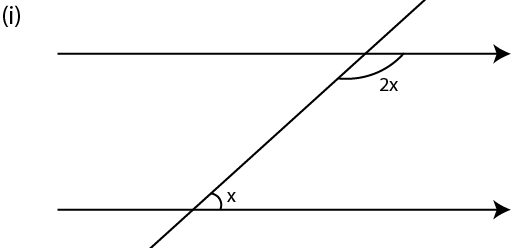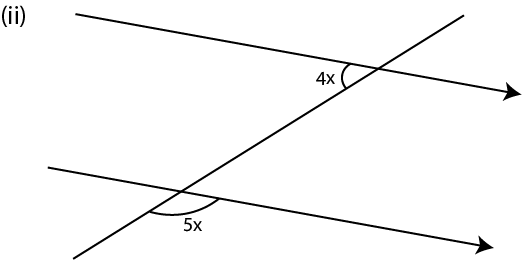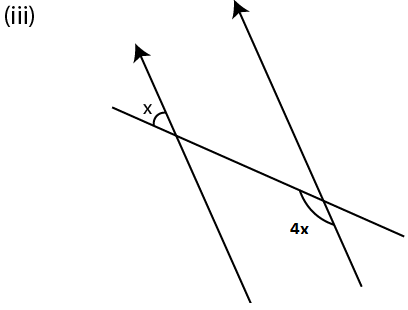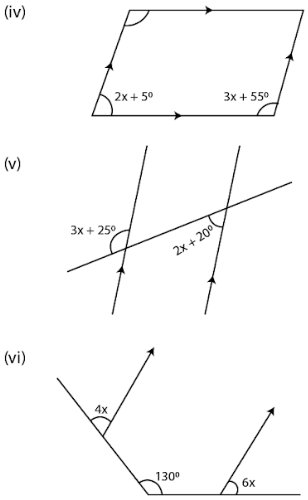Solution:

(i) From the figure (i)

The lines are parallel

2x + x = 1800 are co-interior angles

3x = 1800

x = 180/3 = 600

(ii) From the figure (ii)

The lines are parallel

4x + ∠1 = 1800 are co-interior angles

∠1 = 5x are vertically opposite angles

Substituting the values

4x + 5x = 1800

So we get

9x = 1800

x = 180/9 = 200

(iii) From the figure (iii)

The lines are parallel

∠1 + 4x = 1800 are co-interior angles

∠1 = x are vertically opposite angles

Substituting the values

x + 4x = 1800

5x = 1800

So we get

x = 180/5 = 360

(iv) From the figure (iv)

The lines are parallel

2x + 5 + 3x + 55 = 1800 are co-interior angles

5x + 600 = 1800

By further calculation

5x = 180 – 60 = 1200

So we get

x = 120/5 = 240

(v) From the figure (v)

The lines are parallel

∠1 = 2x + 200 are alternate angles

∠1 + 3x + 250 = 1800 is a linear pair

Substituting the values

2x + 200 + 3x + 250 = 1800

5x + 450 = 1800

So we get

5x = 180 – 45 = 1350

x = 135/5 = 270

(vi) From the figure (vi)

Construct a line parallel to the given parallel lines

∠1 = 4x and ∠2 = 6x are corresponding angles

∠1 + ∠2 = 1300

Substituting the values

4x + 6x = 1300

10x = 1300

So we get

x = 130/10 = 130